## Using H5MD in lammps

H5MD is a HDF5 based file format for molecular data. In short, it is binary, portable and self-describing.

In this blog post I present the use of H5MD in the Molecular Dynamics program lammps. I have written a dump style for lammps, i.e. a piece of code to write the particles' coordinates to a file.

As an example, I present a MD simulation of a Lennard-Jones fluid and a short analysis of the resulting H5MD file. The analysis is deliberately concise to outline the H5MD specifics and not the computations.

Before following this tutorial, you need lammps compiled with the extra package.

I assume that you know how to compile lammps on your platform and add here the bits relevant to H5MD. Obtain lammps:

git clone https://github.com/pdebuyl/lammps.git
cd lammps
git checkout start_dump_h5md



Before compiling lammps itelf you must compile the bundled ch5md library and for this you need HDF5 installed. If it is installed in a standard location you may simply omit the HDF5_PATH optional argument.

cd lib/ch5md
make HDF5_PATH=/path/to/hdf5
cd ../../src
make yes-user-h5md



You may now proceed to your usual lammps compilation.

The input file for lammps is given here. Put it in a file in.lj.

# 3d Lennard-Jones fluid

# Setting a 3D periodic system with lj units
units       lj
dimension   3
atom_style  atomic
boundary p p p

# Create the lattice on which initial atoms will be placed
lattice     sc 0.8
region      box_region block 0 20 0 20 0 20
create_box  1 box_region

# Create the atoms (on the lattice)
create_atoms    1 box
# set their mass to 1
mass        1 1.0

velocity    all create 1.0 79292

pair_style  lj/cut 3.
pair_coeff  1 1 1.0 1.0 3.
pair_modify shift yes

neighbor    0.5 bin
neigh_modify delay 5

fix     1 all nvt temp 1.0 1.0 25.

thermo_style custom time temp ke evdwl press vol density
thermo      100

run 10000

unfix 1
fix 1 all nve
run 10000

dump hd1 all h5md 200 dump_lj.h5 position image author "Pierre de Buyl"

run 20000

Now, run lammps with this input file: /path/to/lmp < in.lj.

Your run directory looks as follows

In :
cd lj_md

/home/pierre/lj_md

In :
ls

dump_lj.h5  in.lj  log.lammps


It is time to analyze the data! As a bonus, I give here a bit of code to parse the logfile generated by lammps and that contains the thermodynamic observables. H5MD can store this data but it is not implemented in lammps yet. Before doing any of the work, let's import a few Python libraries that are available either in your linux distribution or in one of the scientific Python stacks available.

In :
%matplotlib inline
import numpy as np
import matplotlib.pyplot as plt
import h5py
from StringIO import StringIO
plt.rcParams['figure.figsize']= (6,3.6)
plt.rcParams['font.size'] = 18
plt.rcParams['lines.linewidth'] = 2

In :
# Open lammps log file to extract thermodynamic observables
start_indices = [(i,l) for (i,l) in enumerate(logfile) if l.startswith('Time Temp')]
stop_indices = [(i,l) for (i,l) in enumerate(logfile) if l.startswith('Loop time')]
i0 = start_indices[-1]
i1 = stop_indices[-1]
time, temp, e_kin, e_vdw, press, vol, rho = np.loadtxt(StringIO(''.join(logfile[i0+1:i1])), unpack=True)

In :
# Plot a summary of the system evolution
plt.plot(time, e_kin, label='e_kin')
plt.plot(time, e_vdw, label='e_vdw')
plt.legend()
plt.xlabel(r'time');
plt.figure()
plt.plot(time, temp, label='T')
plt.legend()
plt.xlabel(r'time');
print "Average T :", temp.mean()

Average T : 0.981131541542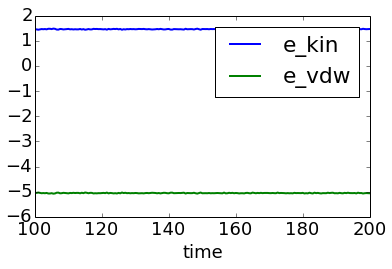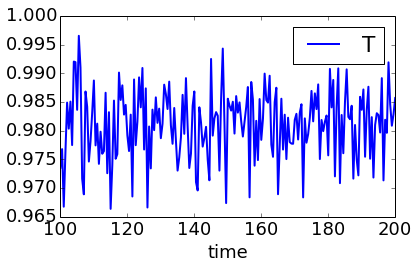## Extracting the coordinates¶

HDF5 files are auto-describing, so you may check their contents. By default, HDF5 ships with a few utilities among which h5ls that allows to list the content of a file.

In :
!h5ls -r dump_lj.h5

/                        Group
/h5md                    Group
/h5md/author             Group
/h5md/creator            Group
/observables             Group
/parameters              Group
/particles               Group
/particles/all           Group
/particles/all/box       Group
/particles/all/box/edges Group
/particles/all/box/edges/step Dataset {101/Inf}
/particles/all/box/edges/time Dataset {101/Inf}
/particles/all/box/edges/value Dataset {101/Inf, 3}
/particles/all/image     Group
/particles/all/image/step Dataset, same as /particles/all/box/edges/step
/particles/all/image/time Dataset, same as /particles/all/box/edges/time
/particles/all/image/value Dataset {101/Inf, 8000, 3}
/particles/all/position  Group
/particles/all/position/step Dataset, same as /particles/all/box/edges/step
/particles/all/position/time Dataset, same as /particles/all/box/edges/time
/particles/all/position/value Dataset {101/Inf, 8000, 3}


A crash course on H5MD is helpful here. The /h5md group contains information on the file (the file format version) and on the creation of the file (author and program).

h5py is an outstanding piece of software to work with HDF5 files. It is used from now on. It has a dict-like interface to access components from HDF5 files.

In :
# First open the file
f = h5py.File('dump_lj.h5', 'r')

# Output the information from the h5md group

print "H5MD file format version  :", f['h5md'].attrs['version']
print "File creator (program)    :", f['h5md/creator'].attrs['name']
print "File creator version      :", f['h5md/creator'].attrs['version']
print "Author of the file        :", f['h5md/author'].attrs['name']

H5MD file format version  : [1 0]
File creator (program)    : lammps
File creator version      : 8 Aug 2014
Author of the file        : Pierre de Buyl


The central data in the file is indeed the particles' trajectories. They are available in /particles/<group_name>/ where <group_name> is chosen by the file's author.

Within the above group, the names are standardised in H5MD. The current file has position, image and box (see above).

In :
position = f['particles/all/position']

print "position['value'].shape :", position['value'].shape

position['value'].shape : (101, 8000, 3)


All groups containing the step, time and value datasets describe data that varies in the course of time. The dimension of value are first the time index (time frames are stacked across this dimension). The second dimension is the particle index. This file contains 8000 particles. The last dimension is the spatial dimension. For each particles, there are x, y and z values.

For instance, get the initial x coordinate of the 17th particle. Then, plot the full trajectory (in z) of the first particle.

In :
print position['value'][0,16,0]

15.6363422409

In :
plt.plot(position['time'], position['value'][:,0,2])
plt.xlabel('time'); plt.ylabel('z for first particle') ;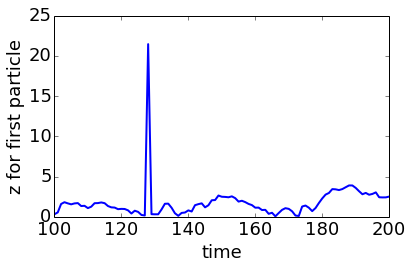The trajectory has a jump across the periodic boundary. This is often the case in molecular dynamics but as we have stored the image data, we may correct for it.

To achieve that result, we also need the box edges and the image coordinates.

In :
box_edges = f['particles/all/box/edges']
image = f['particles/all/image']

In :
plt.plot(position['time'], position['value'][:,0,2]+image['value'][:,0,2]*box_edges['value'][:,2])
plt.xlabel('time'); plt.ylabel('z for first particle') ;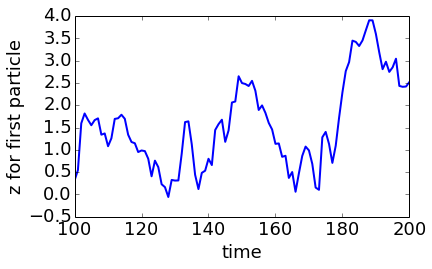Instead of considering the time evolution of a particle, we will now access the data for all particles at a given time step.

In :
from mpl_toolkits.mplot3d import Axes3D
fig = plt.figure()
last_frame = position['value'][-1]
ax.scatter(last_frame[:,0], last_frame[:,1], last_frame[:,2]);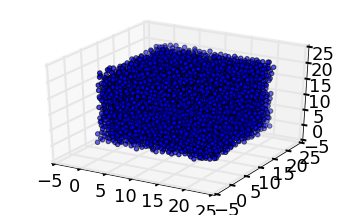To conclude this exploration of a H5MD simulation file, we consider the computation of the radial distribution function.

For this part of the code, I rely on a Cython-based code that found on my github account but that is really not polished for public use. It is a bit more complex than usual because it considers that each particle has a species and a state (that denotes a reactive state). https://github.com/pdebuyl/md_tools

In :
import md_tools._cell_based_rdf

In :
N_points = 100
N_particles = position['value'].shape
species = np.zeros(N_particles, dtype=np.int32)
state = np.zeros(N_particles, dtype=np.int32)
rdf_data = []
for i in range(position['value'].shape)[::10]:
cl = md_tools._cell_based_rdf.CellListRDF(position['value'][i], box_edges['value'][i], 0.8, species, state)
cl.fill()
g, dr, count = cl.rdf_species_state(N_points, 0,0,0,0)
rdf_data.append(g.copy())
r = np.arange(N_points)*dr
rdf_data = np.array(rdf_data)

In :
plt.plot(r[1:], rdf_data.mean(axis=0)[1:]) # The zero-th element of the rdf contains self-contributions and is not relevant
plt.xlabel('r')
plt.ylabel('g(r)');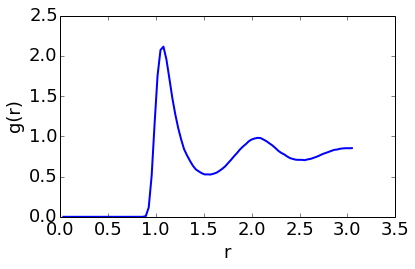In :


In :


In :


In :


In [ ]: